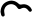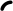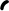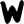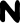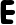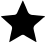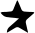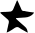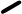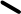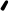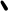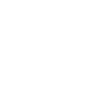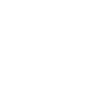O P E R A T I O N H O U R S
Q U I C K L I N K
M O N - F R I : 1 0 A M - 7 P M
S A T - S U N : 1 0 A M - 6 P M
P U B L I C H O L I D A Y : C L O S E D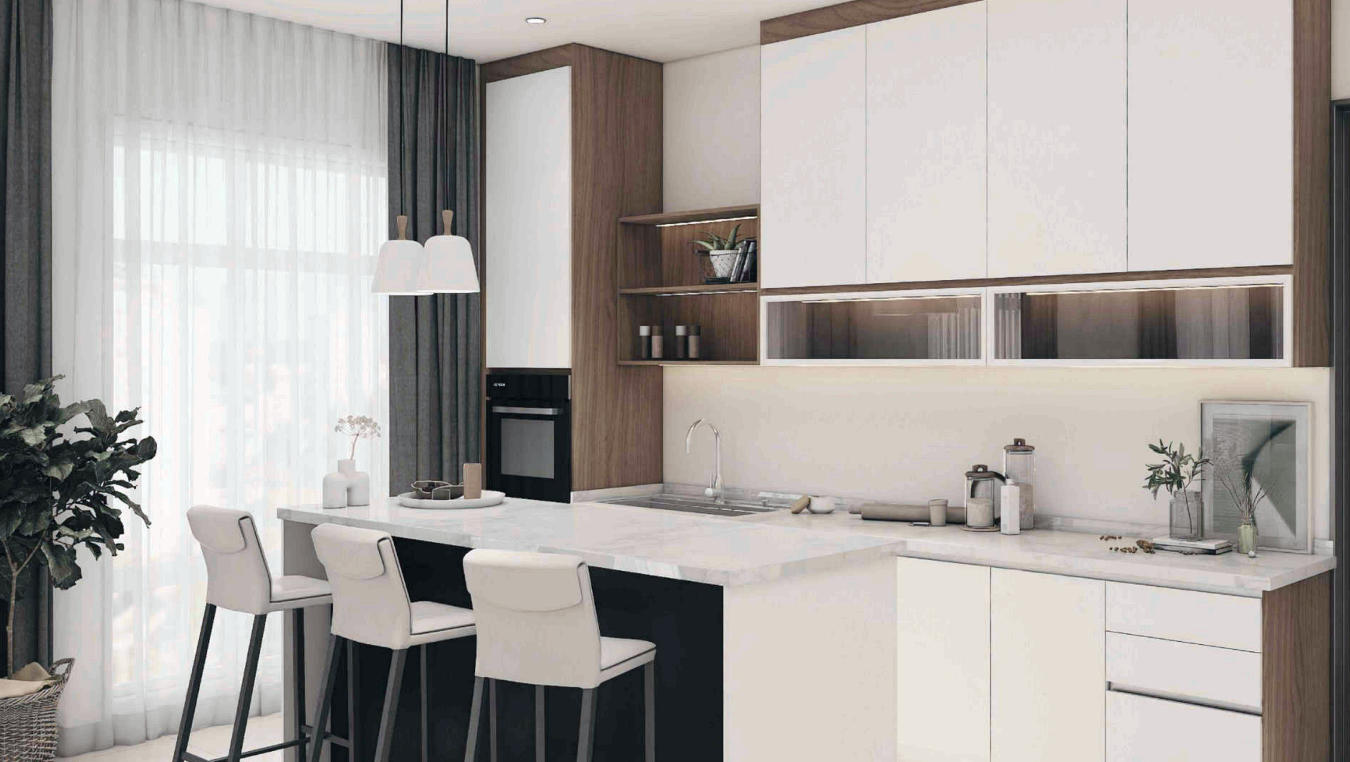# KITCHEN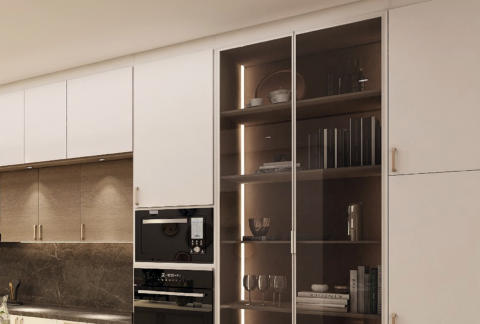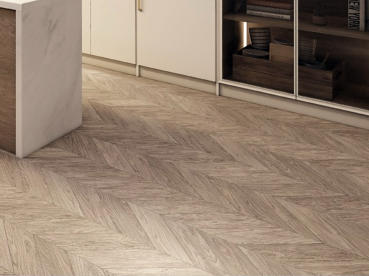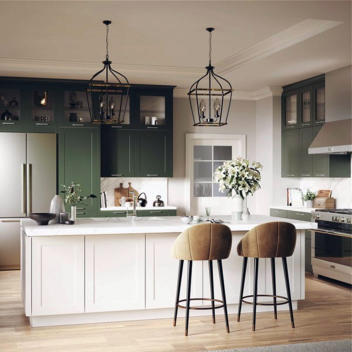# CLASSIC

VIEW MORE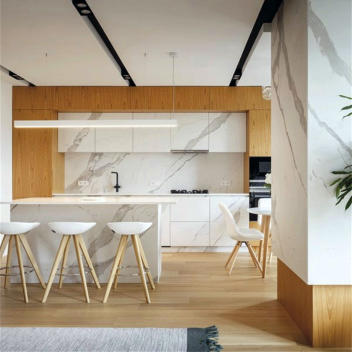# MINIMALISTE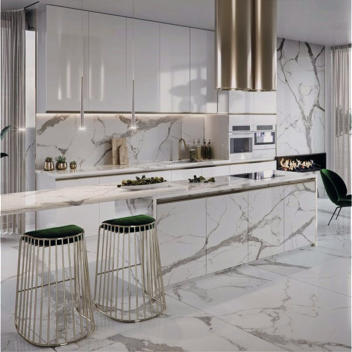# LUXE

VIEW MORE# MODERNE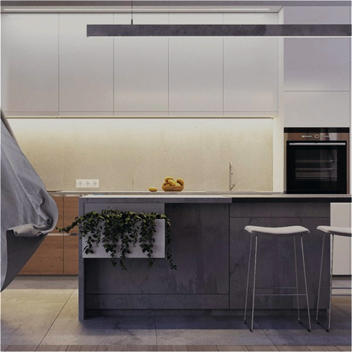# CONTEMPORARYHOMAKITCHEN

# KITCHEN

Homa Kitchen's Premium Series presents kitchen ideas that go beyond feature. This range boasts a balanced mix of types, class, and usefulness, on par with imported European kitchens, thanks to the integration of technology and design trends. Homa Kitchen turns your kitchen into an elegant minimalist canvas, allowing you to express yourself through its diverse compositions. This latest line, created for the urban lifestyle, includes more storage, seamless designs,and integrated lighting and appliances.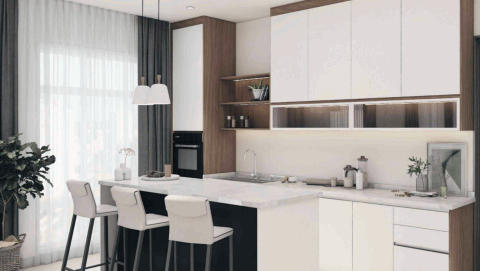O P E R A T I O N H O U R S
M O N - F R I : 1 0 A M - 7 P M
S A T - S U N : 1 0 A M - 6P M
P U B L I C H O L I D A Y : C L O S E D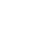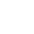HOMAKITCHEN

# KITCHEN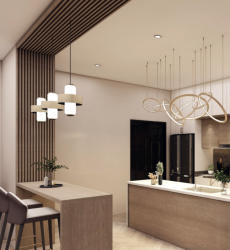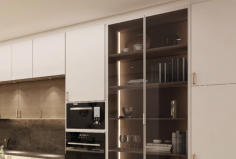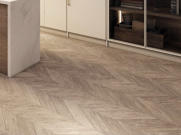# CLASSIC

VIEW MORE# MINIMALISTE# LUXE

VIEW MORE# KITCHEN

Homa Kitchen's Premium Series presents kitchen ideas that go beyond feature. This range boasts a balanced mix of types, class, and usefulness, on par with imported European kitchens, thanks to the integration of technology and design trends. Homa Kitchen turns your kitchen into an elegant minimalist canvas, allowing you to express yourself through its diverse compositions. This latest line, created for the urban lifestyle, includes more storage, seamless designs,and integrated lighting and appliances.# MODERNE

C O N T E M P O R A R Y E U R O P E A N
D E S I G N S & Q U A L I T Y
A W H O L E
N E W D O O R C O L L E C T I O N
I n t e r m s o f e f f i c i e n c y , f u n c t i o n a l i t y , a n d a e s t h e t i c s ,
H O M A K i t c h e n P r e m i u m S e r i e s K i t c h e n S t y l e s c o m p e t e
w i t h E u r o p e a n i m p o r t e d k i t c h e n s . T h e y a r e i n f l u e n c e d
b y t h e c u r r e n t E u r o p e a n d e s i g n t r e n d s .
N e w a n d e x c i t i n g d o o r c o l o u r s a n d s t y l e s
t h a t f o l l o w t h e l a t e s t t r e n d s i n c l u d e a w i d e r a n g e
o f o p t i o n s a n d d e s i g n f l e x i b i l i t y .
C O N T E M P O R A R Y E U R O P E A N
D E S I G N S & Q U A L I T Y
A W H O L E
N E W D O O R C O L L E C T I O N
L o w e r p l i n t h s p r o d u c e a s l i m m e r s h a d o w l i n e ,
r e s u l t i n g i n a s t y l i s h , m o d e r n - l o o k i n g s k i r t i n g w h i l e
s t i l l i n c r e a s i n g s t o r a g e s p a c e .
O u r e x c l u s i v e d a r k g r e y t e x t u r e d c a r c a s s ,
w h i c h i s b u i l t f o r a c l a s s i e r a n d m o r e e l e g a n t s h o w
t h a n a s i m p l e w h i l e c a r c a s s , w i l l
e l e v a t e t h e i n t e r i o r o f y o u r c a b i n e t s .